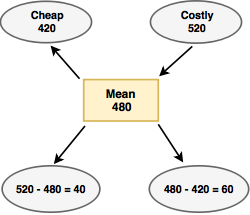# Alligation or Mixture - Aptitude Questions and Answers

## Alligation or Mixture Questions and Answers

Learn and practice the chapter "Alligation or Mixture" with these solved Aptitude Questions and Answers. Each question in the topic is accompanied by a clear and easy explanation, diagrams, formulae, shortcuts and tricks that help in understanding the concept.

## Use of Alligation or Mixture Questions

The questions and examples given in this section will be useful to all the freshers, college students and engineering students preparing for placement tests or any competitive exam like MBA, CAT, MAT, SNAP, MHCET, XAT, NMAT, GATE, Bank exams - IBPS, SBI, RBI, RRB, SSB, SSC, UPSC etc.

Practice with this online test to crack your placements and entrance tests!
1. A shopkeeper mixes two varieties of pulses with price Rs 420/ Kg and Rs 520/ Kg. The price of the mixture he gets is Rs 480/ Kg. Find the ratio of quantities of the two types of pulses to be mixed?

a. 2:3
b. 2:1
c. 1:3
d. 4:1

Explanation:Ratio of Quantities = 40 : 60 = 2:3

## Video : Mixture and Alligation in Hindi - Simple Aptitude tricks for freshers

2. While creating the fragrance mix, a shopkeeper mixes some amount of Jasmine essence with 240 litres water. The price of the mixture is Rs. 275/ litre. The price of Jasmine essence is Rs 325/ litre. What is the quantity of Jasmine essence in the mix?

a. 720 litres
b. 1000 litres
c. 1200 litres
d. 1320 litres

Explanation:Water is free so its price is Rs. 0
Ratio of Water to Essence = 50:275 = 2:11
Water is 2 parts. Hence, 2 parts of water = 240litres.
So 11 parts of essence = ?

 ∴ ? = 11 x 240 = 1320 litres 2

3. An 80L solution of alcohol and water has 45% alcohol in it. If you want the mixture to be 75% alcohol, how much alcohol would you add to it?

a. 30 litres
b. 75 litres
c. 96 litres
d. 110 litres

Explanation:

 Current alcohol quantity = 45 x 80 = 36 Litres 100
 So, 36 + A = 75 x (80+A) 100
∴ A = 96 Litres = This is the additional quantity of alcohol to be added.

4. A vessel contains 40 litres of orange juice. 4 litres of orange juice in the vessel was replaced by water. This process was again repeated twice with the mixture. How much orange juice is there in the final mix?

a. 28 litre
b. 29.16 litre
c. 32 litre
d. 32.29 litre

Explanation:

First 4L orange juice was removed
So now in 40L mixture there is 40 - 4 = 36 litres orange juice and 4 litres water
Now, remove 4L mixture. While doing this proportionate amount of juice and water gets removed.

 Amount of juice removed = 4 x Juice quantity = 4 x 36 = 3.6 Litres Mixture quantity 40
Orange Juice remaining = 36-3.6 = 32.4 Litres
Again 4L mixture removed
 Amount of juice removed = 4 x Juice quantity = 4 x 32.4 = 3.24 Litres Mixture quantity 40
Juice remaining = 32.4-3.24 = 29.16 Litres

5. In a mixture, sugar and jaggery granules are in the ratio 5:3. My mother empties a 16 kg jaggery granules’ bag in this mixture and the new ratio of sugar and jaggery granules becomes 5:7. Find the quantity of jaggery granules in the new mixture?

a. 21 kg
b. 28 kg
c. 35 kg
d. 42 kg